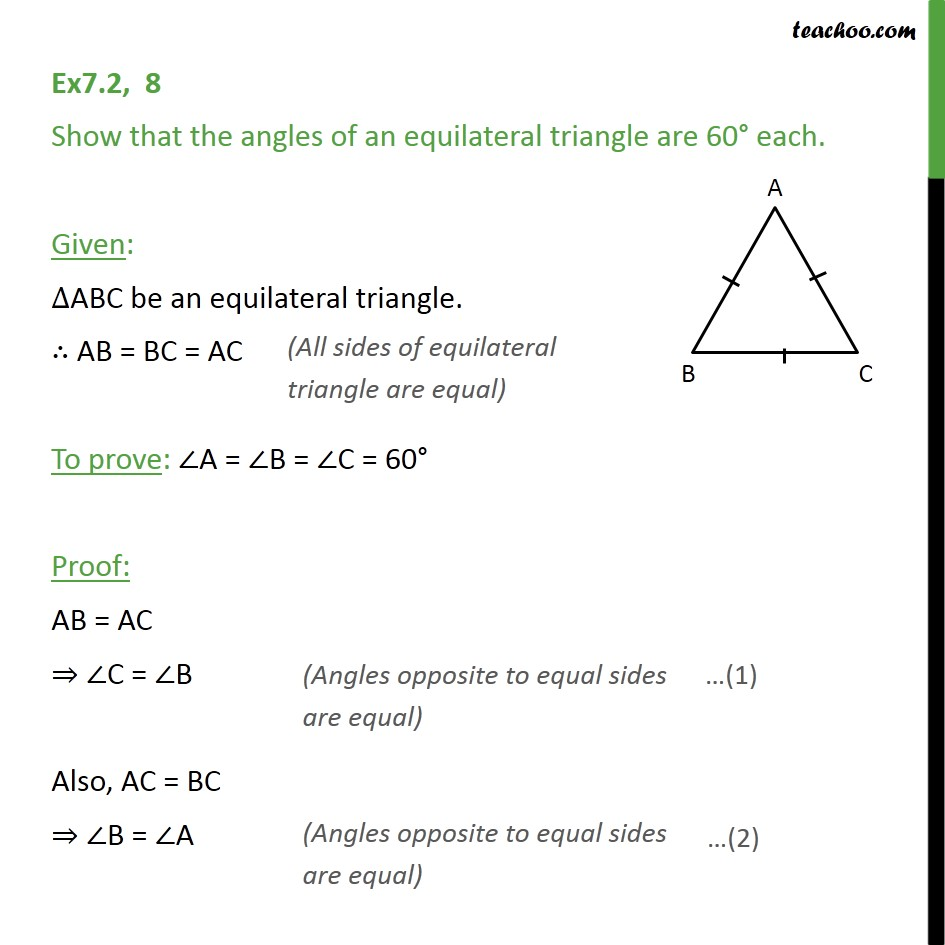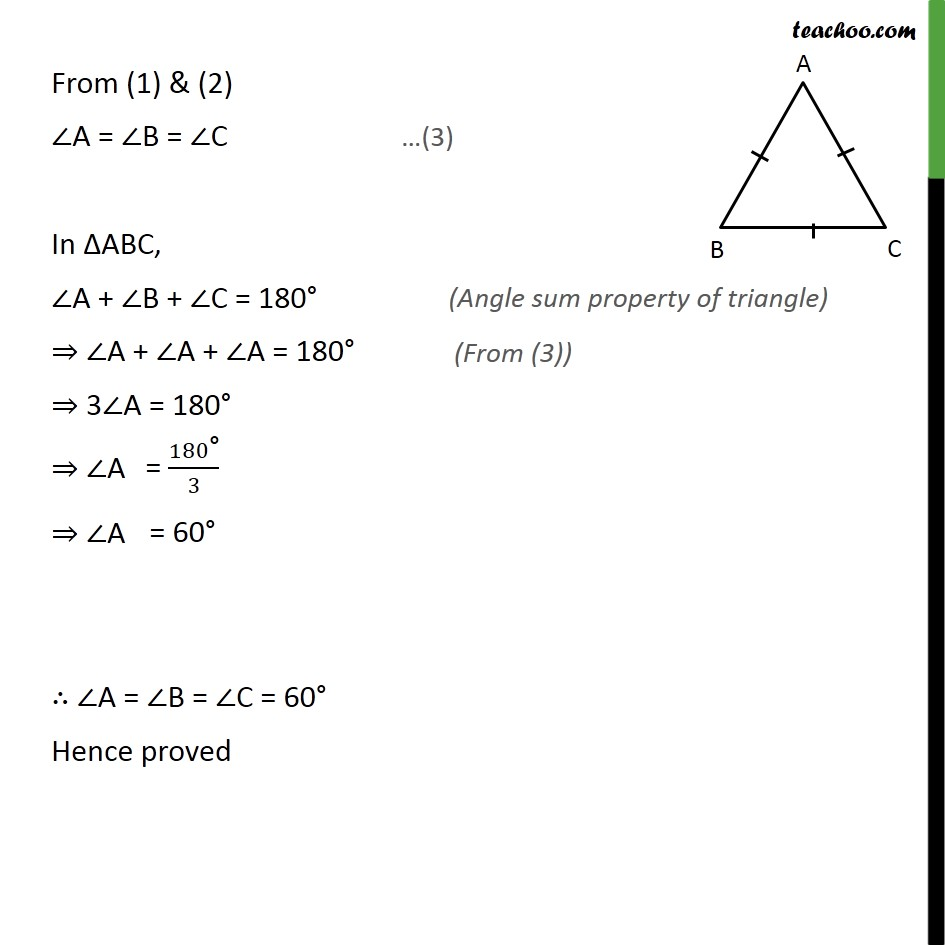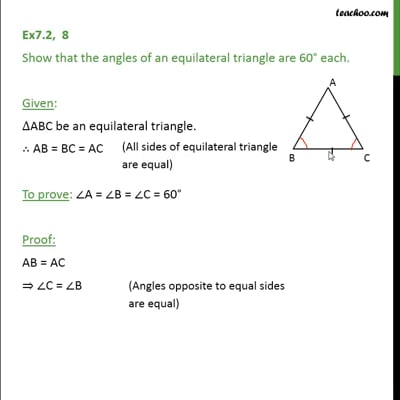Ex 7.2

Chapter 7 Class 9 Triangles
Serial order wiseThis video is only available for Teachoo black users

Maths Crash Course - Live lectures + all videos + Real time Doubt solving!

### Transcript

Ex7.2, 8 Show that the angles of an equilateral triangle are 60 each. Given: ABC be an equilateral triangle. AB = BC = AC To prove: A = B = C = 60 Proof: AB = AC C = B Also, AC = BC B = A From (1) & (2) A = B = C In ABC, A + B + C = 180 A + A + A = 180 3 A = 180 A A A = B = C = 60 Hence proved